# Opamp.pdf

23 de Mar de 2023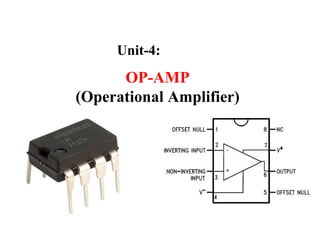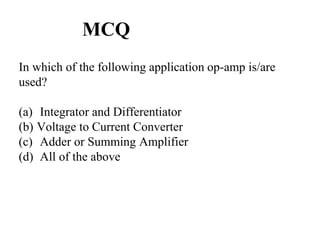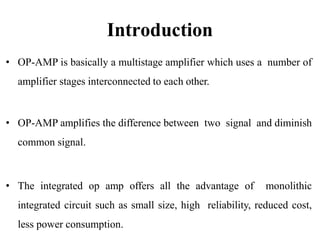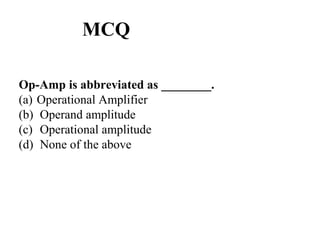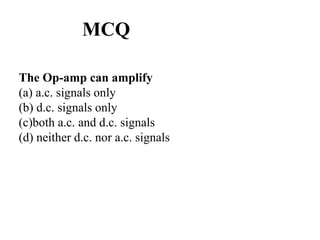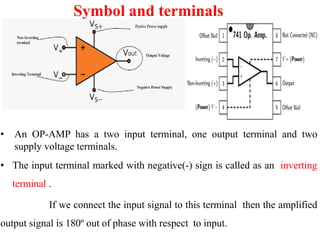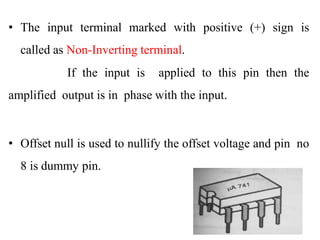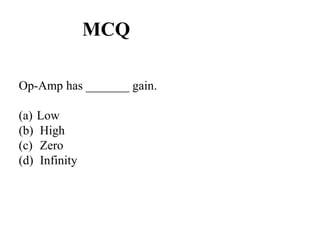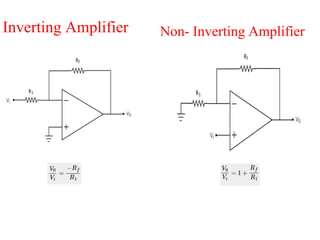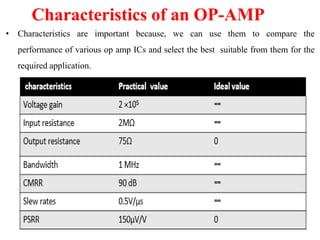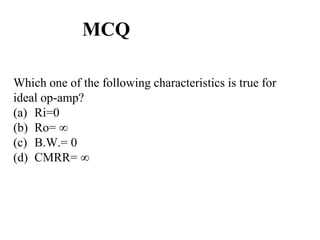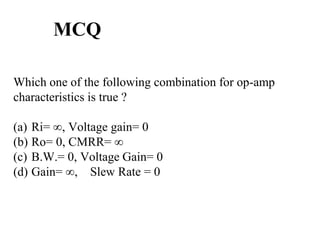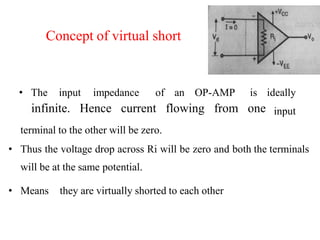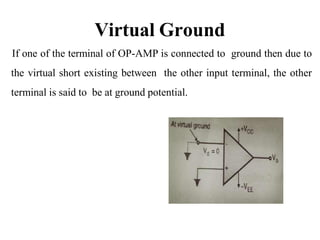1 de 15

### Opamp.pdf

1. OP-AMP (Operational Amplifier) Unit-4:
2. MCQ In which of the following application op-amp is/are used? (a) Integrator and Differentiator (b) Voltage to Current Converter (c) Adder or Summing Amplifier (d) All of the above
3. Introduction • OP-AMP is basically a multistage amplifier which uses a number of amplifier stages interconnected to each other. • OP-AMP amplifies the difference between two signal and diminish common signal. • The integrated op amp offers all the advantage of monolithic integrated circuit such as small size, high reliability, reduced cost, less power consumption.
4. MCQ Op-Amp is abbreviated as ________. (a) Operational Amplifier (b) Operand amplitude (c) Operational amplitude (d) None of the above
5. MCQ The Op-amp can amplify (a) a.c. signals only (b) d.c. signals only (c)both a.c. and d.c. signals (d) neither d.c. nor a.c. signals
6. Symbol and terminals • An OP-AMP has a two input terminal, one output terminal and two supply voltage terminals. • The input terminal marked with negative(-) sign is called as an inverting terminal . If we connect the input signal to this terminal then the amplified output signal is 180º out of phase with respect to input.
7. • The input terminal marked with positive (+) sign is called as Non-Inverting terminal. If the input is applied to this pin then the amplified output is in phase with the input. • Offset null is used to nullify the offset voltage and pin no 8 is dummy pin.
8. MCQ Op-Amp has _______ gain. (a) Low (b) High (c) Zero (d) Infinity
9. Inverting Amplifier Non- Inverting Amplifier
10. Characteristics of an OP-AMP • Characteristics are important because, we can use them to compare the performance of various op amp ICs and select the best suitable from them for the required application.
11. MCQ Which one of the following characteristics is true for ideal op-amp? (a) Ri=0 (b) Ro= ∞ (c) B.W.= 0 (d) CMRR= ∞
12. MCQ Which one of the following characteristics is not true for ideal op-amp? (a) Ri= ∞ (b) Ro= 0 (c) B.W.= 0 (d) Gain= ∞
13. MCQ Which one of the following combination for op-amp characteristics is true ? (a) Ri= ∞, Voltage gain= 0 (b) Ro= 0, CMRR= ∞ (c) B.W.= 0, Voltage Gain= 0 (d) Gain= ∞, Slew Rate = 0
14. infinite. Hence current flowing from one • The input impedance of an OP-AMP is ideally input terminal to the other will be zero. • Thus the voltage drop across Ri will be zero and both the terminals will be at the same potential. • Means they are virtually shorted to each other Concept of virtual short
15. Virtual Ground If one of the terminal of OP-AMP is connected to ground then due to the virtual short existing between the other input terminal, the other terminal is said to be at ground potential.